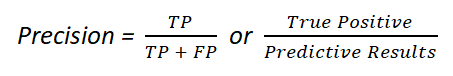top of pageSearch

# What is a Confusion Matrix in Machine Learning?

Image source

A Confusion matrix is an N x N matrix used for evaluating the performance of a classification model, where N is the number of target classes. The matrix compares the actual target values with those predicted by the machine learning model.

For a binary classification, the confusion matrix is 2 x 2 as shown below with 4 output:A binary classifier predicts all data instances of a test data set as either positive or negative. This produces four outcomes-

1. True-positive(TP): TP is correct positive prediction means that the actual value was positive and the model predicted a positive value.

2. False-positive(FP): FP is incorrect positive prediction means that the actual value was negative but the model predicted a positive value. It is also known as the Type 1 error.

3. True-negative(TN): TN is correct negative prediction means that the actual value was negative and the model predicted a negative value.

4. False-negative(FN): FN is incorrect negative prediction means that the actual value was positive but the model predicted a negative value. It is also known as the Type 2 error.

### Basic measures derived from the confusion matrix

Accuracy: Classification accuracy is the ratio of correct predictions to total predictions made.Sensitivity(Recall or True positive rate): It is also called recall (REC) or true positive rate (TPR). Sensitivity is calculated as the number of correct positive predictions(TP) divided by the total number of positives.Specificity(True negative rate): It is also called true negative rate (TNR). Specificity is calculated as the number of correct negative predictions (TN) divided by the total number of negatives.Precision(Positive predicted value): Precision means false positives are cases the model incorrectly labels as positive that are actually negative, or for example, individuals the model classifies as terrorists that are not.Error Rate: It is calculated as the number of all incorrect predictions divided by the total number of the dataset. The best error rate is 0.0, whereas the worst is 1.0.F-Score or F1-Score(Harmonic mean of precision and recall): F Score is the weighted average of Precision and Recall. Therefore, this score takes both false positives and false negatives into account. Intuitively it is not as easy to understand as accuracy, but F is usually more useful than accuracy, especially if you have an uneven class distribution. Accuracy works best if false positives and false negatives have similar cost. If the cost of false positives and false negatives are very different, it’s better to look at both Precision and Recall.Thank you.